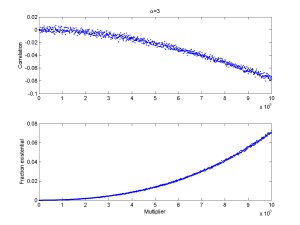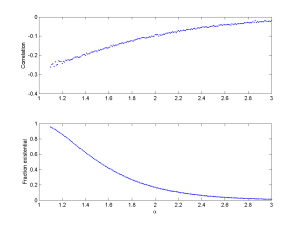# Anthropic negativesStuart Armstrong has come up with another twist on the anthropic shadow phenomenon. If existential risk needs two kinds of disasters to coincide in order to kill everybody, then observers will notice the disaster types to be anticorrelated.

The minimal example would be if each risk had 50% independent chance of happening: then the observable correlation coefficient would be -0.5 (not -1, since there is 1/3 chance to get neither risk; the possible outcomes are: no event, risk A, and risk B). If the probability of no disaster happening is N/(N+2) and the risks are equal 1/(N+2), then the correlation will be -1/(N+1).

I tried a slightly more elaborate model. Assume X and Y to be independent power-law distributed disasters (say war and pestillence outbreaks), and that if X+Y is larger than seven billion no observers will remain to see the outcome. If we ramp up their size (by multiplying X and Y with some constant) we get the following behaviour (for alpha=3):(Top) correlation between observed power-law distributed independent variables multiplied by an increasing multiplier, where observation is contingent on their sum being smaller than 7 billion. Each point corresponds to 100,000 trials. (Bottom) Fraction of trials where observers were wiped out.

As the situation gets more deadly the correlation becomes more negative. This also happens when allowing the exponent run from the very fat (alpha=1) to the thinner (alpha=3):(top) Correlation between observed independent power-law distributed variables (where observability requires their sum to be smaller than seven billion) for different exponents. (Bottom) fraction of trials ending in existential disaster. Multiplier=500 million.

The same thing also happens if we multiply X and Y.

I like the phenomenon: it gives us a way to look for anthropic effects by looking for suspicious anticorrelations. In particular, for the same variable the correlation ought to shift from near zero for small cases to negative for large cases. One prediction might be that periods of high superpower tension would be anticorrelated with mishaps in the nuclear weapon control systems. Of course, getting the data might be another matter. We might start by looking at extant companies with multiple risk factors like insurance companies and see if capital risk becomes anticorrelated with insurance risk at the high end.